# Gabor transform

(diff) ← Older revision | Latest revision (diff) | Newer revision → (diff)

An integral transform introduced by D. Gabor, the Hungarian-born Nobel laureate in physics, who, in his paper [a3], modified the well-known Fourier transform of a function (or a signal)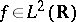by introducing a time-localization window function (also called a time-frequency window). Let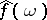denote the Fourier transform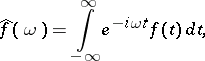and let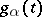denote the Gaussian function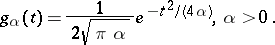Then the Gabor transform ofis defined by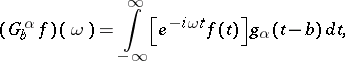where the real parameteris used to translate the "window". The Gabor transform localizes the Fourier transform at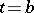. A similar transform can be introduced for Fourier series.

By choosing more general windows, the transforms are called short-time Fourier transform and the Gabor transform is a special case, based on the Gaussian window. One property of the special choiceis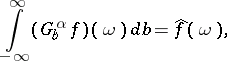which says that the set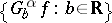of Gabor transforms ofdecomposes the Fourier transform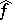ofexactly.

Gabor transforms (and related topics based on the Gabor transform) are applied in numerous engineering applications, many of them without obvious connection to the traditional field of time-frequency analysis for deterministic signals. Detailed information (including many references) about the use of Gabor transforms in such diverse fields as image analysis, object recognition, optics, filter banks, or signal detection can be found in [a4], the first book devoted to Gabor transforms and related analysis.

A recent development (starting at 1992) that is more effective for analyzing signals with sharp variations is based on wavelets (see [a2] or Wavelet analysis); for the relation between wavelets and the Gabor transform, see [a1]. The Gabor transform can also be viewed in connection with "coherent states" associated with the Weyl–Heisenberg group; see [a4].

How to Cite This Entry:
Gabor transform. Encyclopedia of Mathematics. URL: http://encyclopediaofmath.org/index.php?title=Gabor_transform&oldid=11937
This article was adapted from an original article by N.M. Temme (originator), which appeared in Encyclopedia of Mathematics - ISBN 1402006098. See original article